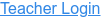### Topics

• Slope-Intercept Form
• Graphing Linear Equations

### Description

Explore slope-intercept form of a line. Connect the slope and y-intercept to the equation of the line. Challenge yourself in the line game!

### Sample Learning Goals

• Graph a line given an equation in slope-intercept form.
• Write an equation in slope-intercept form given a graphed line.
• Predict how changing the values in a linear equation will affect the graphed line.
• Predict how changing the graphed line will affect the equation.
Version 1.0.2